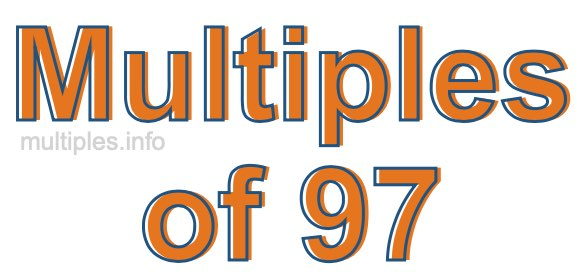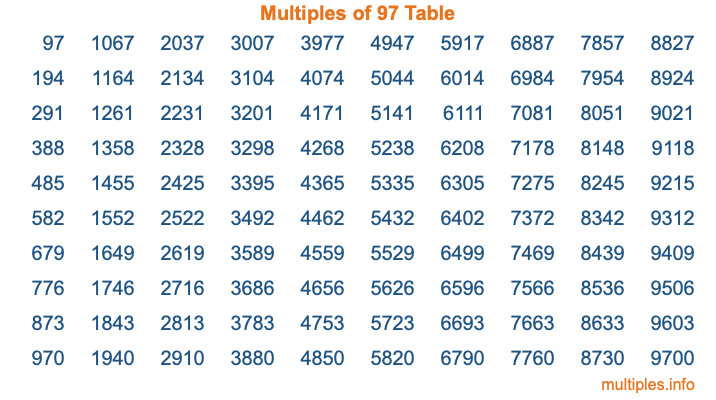Multiples of 97Welcome to the Multiples of 97 page. Here we will first teach you everything you will ever need to know about the multiples of 97, and then give you a study guide summary of everything we taught you to make sure you remember it all. Use this page to look up facts and learn information about the multiples of 97. This page will make you a multiples of ninety-seven expert!

Definition of Multiples of 97
Multiples of 97 are all the numbers that when divided by 97 equal an integer. Each of the multiples of 97 are called a multiple. A multiple of 97 is created by multiplying 97 by an integer.

Therefore, to create a list of multiples of 97, you start with 1 multiplied by 97, then 2 multiplied by 97, then 3 multiplied by 97, and so on for as long as you want. Thus, the list of the first five multiples of 97 is 97, 194, 291, 388, and 485. To see a larger list of multiples of 97, see the printable image of Multiples of 97 further down on this page. We also have a category where you can choose any nth multiple of 97.

Multiples of 97 Checker
The Multiples of 97 Checker below checks to see if any number of your choice is a multiple of 97. In other words, it checks to see if there is any number (integer) that when multiplied by 97 will equal your number. To do that, we divide your number by 97. If the the quotient is an integer, then your number is a multiple of 97.

Is  a multiple of 97?

Least Common Multiple of 97 and ...
A Least Common Multiple (LCM) is the lowest multiple that two or more numbers have in common. This is also called the smallest common multiple or lowest common multiple and is useful to know when you are adding our subtracting fractions. Enter one or more numbers below (97 is already entered) to find the LCM.

Check out our LCM Calculator if you need more details about the Least Common Multiple or if you need the LCM for different numbers for adding and subtraction fractions.

nth Multiple of 97
As we stated above, 97 is the first multiple of 97, 194 is the second multiple of 97, 291 is the third multiple of 97, and so on. Enter a number below to find the nth multiple of 97.

th multiple of 97

Multiples of 97 vs Factors of 97
97 is a multiple of 97 and a factor of 97, but that is where the similarities end. All postive multiples of 97 are 97 or greater than 97. All positive factors of 97 are 97 or less than 97.

Below is the beginning list of multiples of 97 and the factors of 97 so you can compare:

Multiples of 97: 97, 194, 291, 388, 485, etc.

Factors of 97: 1, 97

As you can see, the multiples of 97 are all the numbers that you can divide by 97 to get a whole number. The factors of 97, on the other hand, are all the whole numbers that you can multiply by another whole number to get 97.

It's also interesting to note that if a number (x) is a factor of 97, then 97 will also be a multiple of that number (x).

Multiples of 97 vs Divisors of 97
The divisors of 97 are all the integers that 97 can be divided by evenly. Below is a list of the divisors of 97.

Divisors of 97: 1, 97

The interesting thing to note here is that if you take any multiple of 97 and divide it by a divisor of 97, you will see that the quotient is an integer.

Multiples of 97 Table
Below is an image of the first 100 multiples of 97 in a table. The table is in chronological order, column by column. The first column has the first ten multiples of 97, the second column has the next ten multiples of 97, and so on.The Multiples of 97 Table is also referred to as the 97 Times Table or Times Table of 97. You are welcome to print out our table for your studies.

Negative Multiples of 97
Although not often discussed or needed in math, it is worth mentioning that you can make a list of negative multiples of 97 by multiplying 97 by -1, then by -2, then by -3, and so on, to get the following list of negative multiples of 97:

-97, -194, -291, -388, -485, etc.

Multiples of 97 Summary
Below is a summary of important Multiples of 97 facts that we have discussed on this page. To retain the knowledge on this page, we recommend that you read through the summary and explain to yourself or a study partner why they hold true.

There are an infinite number of multiples of 97.

A multiple of 97 divided by 97 will equal a whole number.

97 divided by a factor of 97 equals a divisor of 97.

The nth multiple of 97 is n times 97.

The largest factor of 97 is equal to the first positive multiple of 97.

97 is a multiple of every factor of 97.

97 is a multiple of 97.

A multiple of 97 divided by a divisor of 97 equals an integer.

97 divided by a divisor of 97 equals a factor of 97.

Any integer times 97 will equal a multiple of 97.

Multiples of a Number
Here you can get the multiples of another number, all with the same attention to detail as we did for multiples of 97 on this page.

Multiples of
Multiples of 98
Did you find our page about multiples of ninety-seven educational? Do you want more knowledge? Check out the multiples of the next number on our list!